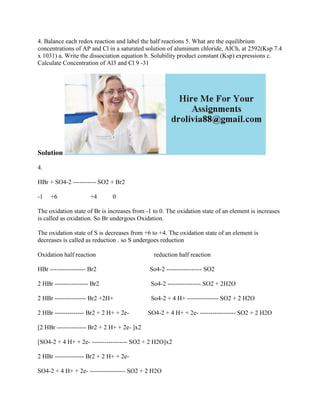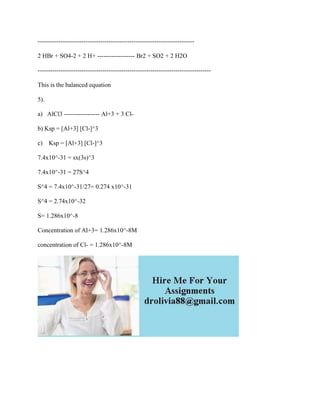Se está descargando tu SlideShare. ×

# 4- Balance each redox reaction and label the half reactions 5- What ar.docx

Anuncio
Anuncio
Anuncio
Anuncio
Anuncio
Anuncio
Anuncio
Anuncio
Anuncio
Anuncio
AnuncioCargando en…3
×

1 de 2 Anuncio

# 4- Balance each redox reaction and label the half reactions 5- What ar.docx

4. Balance each redox reaction and label the half reactions 5. What are the equilibrium concentrations of AP and Cl in a saturated solution of aluminum chloride, AICh, at 2592(Ksp 7.4 x 1031) a. Write the dissociation equation b. Solubility product constant (Ksp) expressions c. Calculate Concentration of Al3 and Cl 9 -31
Solution
4.
HBr + SO4-2 ----------- SO2 + Br2
-1     +6                     +4          0
The oxidation state of Br is increases from -1 to 0. The oxidation state of an element is increases is called as oxidation. So Br undergoes Oxidation.
The oxidation state of S is decreases from +6 to +4. The oxidation state of an element is decreases is called as reduction . so S undergoes reduction
Oxidation half reaction                                     reduction half reaction
HBr ----------------- Br2                                  So4-2 ----------------- SO2
2 HBr ---------------- Br2                                 So4-2 ---------------- SO2 + 2H2O
2 HBr --------------- Br2 +2H+                        So4-2 + 4 H+ --------------- SO2 + 2 H2O
2 HBr -------------- Br2 + 2 H+ + 2e-            SO4-2 + 4 H+ + 2e- ----------------- SO2 + 2 H2O
[2 HBr -------------- Br2 + 2 H+ + 2e- ]x2
[SO4-2 + 4 H+ + 2e- ----------------- SO2 + 2 H2O]x2
2 HBr -------------- Br2 + 2 H+ + 2e-
SO4-2 + 4 H+ + 2e- ----------------- SO2 + 2 H2O
---------------------------------------------------------------------------
2 HBr + SO4-2 + 2 H+ ------------------ Br2 + SO2 + 2 H2O
-----------------------------------------------------------------------------------
This is the balanced equation
5).
a)   AlCl3 ----------------- Al+3 + 3 Cl-
b) Ksp = [Al+3] [Cl-]^3
c)    Ksp = [Al+3] [Cl-]^3
7.4x10^-31 = sx(3s)^3
7.4x10^-31 = 27S^4
S^4 = 7.4x10^-31/27= 0.274 x10^-31
S^4 = 2.74x10^-32
S= 1.286x10^-8
Concentration of Al+3= 1.286x10^-8M
concentration of Cl- = 1.286x10^-8M
.

4. Balance each redox reaction and label the half reactions 5. What are the equilibrium concentrations of AP and Cl in a saturated solution of aluminum chloride, AICh, at 2592(Ksp 7.4 x 1031) a. Write the dissociation equation b. Solubility product constant (Ksp) expressions c. Calculate Concentration of Al3 and Cl 9 -31
Solution
4.
HBr + SO4-2 ----------- SO2 + Br2
-1     +6                     +4          0
The oxidation state of Br is increases from -1 to 0. The oxidation state of an element is increases is called as oxidation. So Br undergoes Oxidation.
The oxidation state of S is decreases from +6 to +4. The oxidation state of an element is decreases is called as reduction . so S undergoes reduction
Oxidation half reaction                                     reduction half reaction
HBr ----------------- Br2                                  So4-2 ----------------- SO2
2 HBr ---------------- Br2                                 So4-2 ---------------- SO2 + 2H2O
2 HBr --------------- Br2 +2H+                        So4-2 + 4 H+ --------------- SO2 + 2 H2O
2 HBr -------------- Br2 + 2 H+ + 2e-            SO4-2 + 4 H+ + 2e- ----------------- SO2 + 2 H2O
[2 HBr -------------- Br2 + 2 H+ + 2e- ]x2
[SO4-2 + 4 H+ + 2e- ----------------- SO2 + 2 H2O]x2
2 HBr -------------- Br2 + 2 H+ + 2e-
SO4-2 + 4 H+ + 2e- ----------------- SO2 + 2 H2O
---------------------------------------------------------------------------
2 HBr + SO4-2 + 2 H+ ------------------ Br2 + SO2 + 2 H2O
-----------------------------------------------------------------------------------
This is the balanced equation
5).
a)   AlCl3 ----------------- Al+3 + 3 Cl-
b) Ksp = [Al+3] [Cl-]^3
c)    Ksp = [Al+3] [Cl-]^3
7.4x10^-31 = sx(3s)^3
7.4x10^-31 = 27S^4
S^4 = 7.4x10^-31/27= 0.274 x10^-31
S^4 = 2.74x10^-32
S= 1.286x10^-8
Concentration of Al+3= 1.286x10^-8M
concentration of Cl- = 1.286x10^-8M
.

Anuncio
Anuncio

### 4- Balance each redox reaction and label the half reactions 5- What ar.docx

1. 1. 4. Balance each redox reaction and label the half reactions 5. What are the equilibrium concentrations of AP and Cl in a saturated solution of aluminum chloride, AICh, at 2592(Ksp 7.4 x 1031) a. Write the dissociation equation b. Solubility product constant (Ksp) expressions c. Calculate Concentration of Al3 and Cl 9 -31 Solution 4. HBr + SO4-2 ----------- SO2 + Br2 -1 +6 +4 0 The oxidation state of Br is increases from -1 to 0. The oxidation state of an element is increases is called as oxidation. So Br undergoes Oxidation. The oxidation state of S is decreases from +6 to +4. The oxidation state of an element is decreases is called as reduction . so S undergoes reduction Oxidation half reaction reduction half reaction HBr ----------------- Br2 So4-2 ----------------- SO2 2 HBr ---------------- Br2 So4-2 ---------------- SO2 + 2H2O 2 HBr --------------- Br2 +2H+ So4-2 + 4 H+ --------------- SO2 + 2 H2O 2 HBr -------------- Br2 + 2 H+ + 2e- SO4-2 + 4 H+ + 2e- ----------------- SO2 + 2 H2O [2 HBr -------------- Br2 + 2 H+ + 2e- ]x2 [SO4-2 + 4 H+ + 2e- ----------------- SO2 + 2 H2O]x2 2 HBr -------------- Br2 + 2 H+ + 2e- SO4-2 + 4 H+ + 2e- ----------------- SO2 + 2 H2O
2. 2. --------------------------------------------------------------------------- 2 HBr + SO4-2 + 2 H+ ------------------ Br2 + SO2 + 2 H2O ----------------------------------------------------------------------------------- This is the balanced equation 5). a) AlCl3 ----------------- Al+3 + 3 Cl- b) Ksp = [Al+3] [Cl-]^3 c) Ksp = [Al+3] [Cl-]^3 7.4x10^-31 = sx(3s)^3 7.4x10^-31 = 27S^4 S^4 = 7.4x10^-31/27= 0.274 x10^-31 S^4 = 2.74x10^-32 S= 1.286x10^-8 Concentration of Al+3= 1.286x10^-8M concentration of Cl- = 1.286x10^-8M